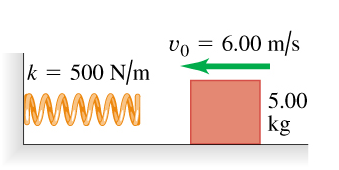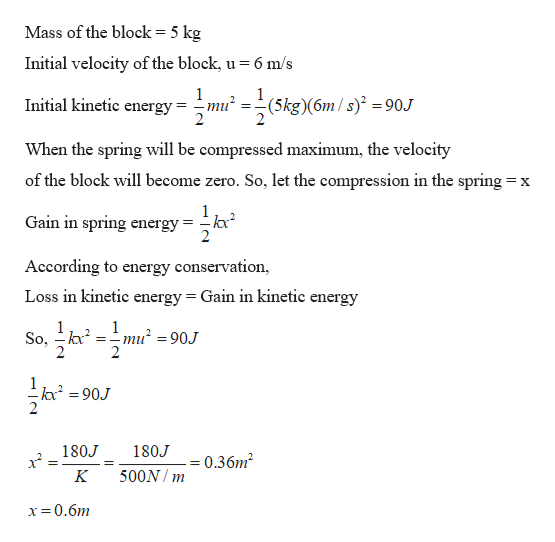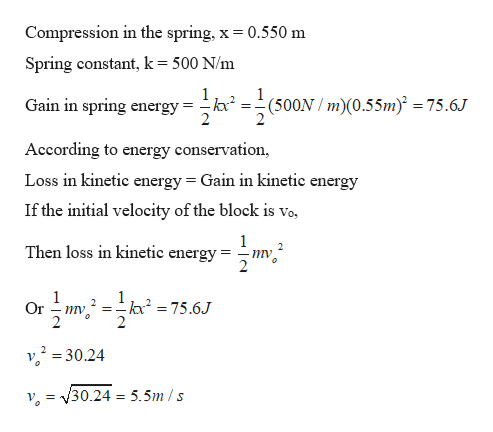Question
838 views

A 5.00-kg block is moving at 6.00 m/s along a frictionless, horizontal surface toward a spring with force constant k=500 N/m that is attached to a wall (the figure (Figure 1)). The spring has negligible mass.

Find the maximum distance the spring will be compressed.
If the spring is to compress by no more than 0.550 m , what should be the maximum value of v0?help_outlineImage Transcriptionclosevo 6.00 m/s k = 500 N/m 5.00 kg fullscreen
check_circle

Step 1

Given:

Mass of the block = 5 kg

Velocity of the block = 6 m/s

Spring constant = 500 N/m

Step 2

Calculating the maximum compression in the spring:help_outlineImage TranscriptioncloseMass of the block = 5 kg Initial velocity of the block, u 6 m/s 1 (5kg)(6m/s) 90J 2 Initial kinetic energy When the spring will be compressed maximum, the velocity of the block will become zero. So, let the compression in the spring = X 1 Gain in spring energy 2 According to energy conservation Loss in kinetic energy = Gain in kinetic energy 1 So, 1 mu2 =90J 2 kc2 90J 180J 180J = 0.36m2 500N m K x0.6m fullscreen
Step 3

Finding the required velo...help_outlineImage TranscriptioncloseCompression in the spring, x = 0.550 m Spring constant, k = 500 N/m 1 Gain in spring energy 2 1 -(500N m)(0.55m) = 75.6J 2 According to energy conservation Loss in kinetic energy Gain in kinetic energy If the initial velocity of the block is Vo, 1 Then loss in kinetic energy = тy 1 ka2 75.6J Or ту. v=30.24 V V=V30.24 = 5.5m /s fullscreen

### Want to see the full answer?

See Solution

#### Want to see this answer and more?

Solutions are written by subject experts who are available 24/7. Questions are typically answered within 1 hour.*

See Solution
*Response times may vary by subject and question.
Tagged in

### Work,Power and Energy